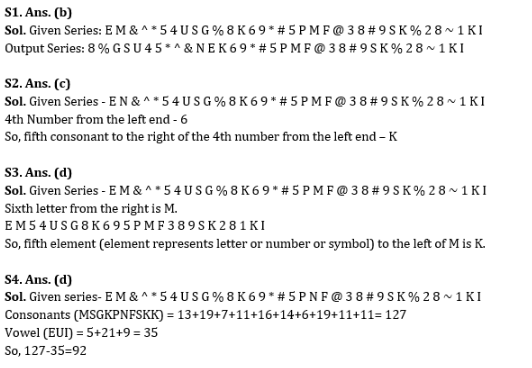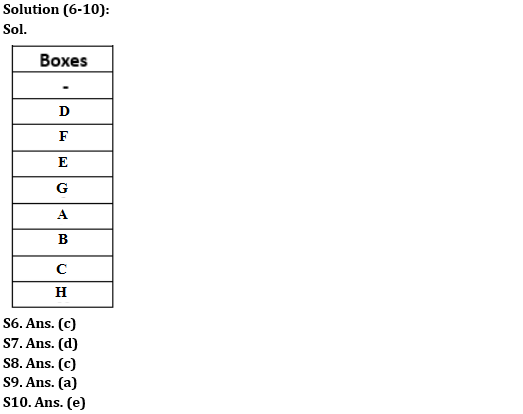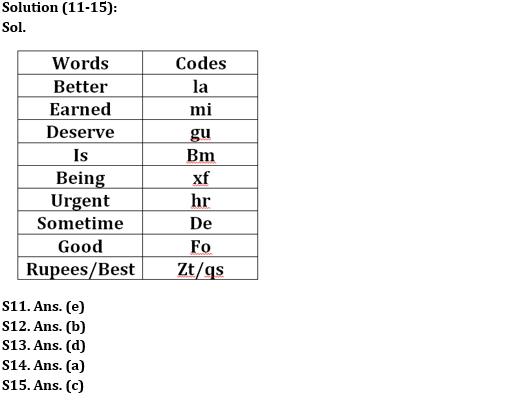Latest Banking jobs   »

# Reasoning Ability Quiz For SBI Clerk Prelims 2022- 20ty May

Directions (1-5): The given questions are based on the following arrangement of letter, numbers and symbols.

E M & ^ * 5 4 U S G % 8 K 6 9 * # 5 P N F @ 3 8 # 9 S K % 2 8 ~ 1 K I

Q1. If the first twelve elements are reversed then which letter is eighth to the left of the seventh element from the right end?
(a) P
(b) F
(c) M
(d) Q
(e) None of these

Q2. Which is the fifth consonant to the right of the 4th number from the left end in the arrangement?
(a) P
(b) F
(c) K
(d) S
(e) None of these

Q3. If all the symbols are dropped from the series, then which of the following element is fifth to the left of the sixth letter from the right end?
(a) G
(b) 6
(c) 8
(d) K
(e) None of these

Q4. If we add the place value of all consonants and subtract it from the addition of all vowel’s place value then what will be the final value?
(a) 90
(b) 80
(c) 82
(d) 92
(e) None of these

Q5. Which element is second to the right of fifth element from the right end?
(a) S
(b) K
(c) F
(d) 1
(e) None of these

Directions (6-10): Study the following information carefully and answer the given questions.
Eight boxes A, B, C, D, E, F, G and H are arranged in a stack from top to bottom but not necessarily in the same order and one vacant stack is also between them. Box H is kept either at the top or bottom of the stack. Two boxes are kept between Box H and A. Vacant box does not lie between Box H and Box A. B is kept immediately below Box A. Two boxes are kept between Box B and E where Box E is kept above Box B. Number of boxes kept between Box E and Box A is same as the number of boxes kept between Box A and Box C. Box D is kept somewhere above Box C but it is not kept adjacent to Box E. Box D does not place above vacant stack. Box F is kept above Box G but below vacant stack.

Q6. Which amongst the following boxes are kept at the top and bottom of the stack respectively?
(a) Box H and Vacant box
(b) Box H and Box A
(c) Vacant box and Box H
(d) Box H and Box D
(e) None of these

Q7. How many boxes are kept above Box C?
(a) More than six
(b) Five
(c) Four
(d) Six
(e) None of these

Q8. As many boxes are kept between Box C and Box G as between Box A and ____?
(a) Box F
(b) Box H
(c) Both Box F and Box H
(d) Box E
(e) None of these

Q9. Which box is kept immediately above Box G?
(a) Box E
(b) Box D
(c) Box C
(d) Box H
(e) None of these

Q10. Which of the following is/are true with respect to the given arrangement?
1) Box C is kept immediately below Box B.
2) Box D is kept at the top of the stack.
3) Only one box is kept between Box D and Box E.

(a) Both (1) and (2)
(b) Only (2)
(c) Only (1)
(d) All (1), (2) and (3)
(e) Both (1) and (3)

Directions (11-15): Study the following information carefully and answer the questions given.
In a certain code language,
‘Good better earned deserve’ is written as ‘fo mi la gu’;
‘Deserve is sometime earned’ is written as ‘de gu mi bm’;
‘Better being is urgent’ is written as ‘bm hr la xf’;
‘Earned rupees being best’ is written as ‘zt qs mi xf’.

Q11. Which of the following will be the possible code for ‘rupees’?
(a) zt
(b) qs
(c) mi
(d) xf
(e) zt or qs

Q12. In the given code language ‘good urgent’ can be written as _____?
(a) fo la
(b) hr fo
(c) hr bm
(d) gu la
(e) fo de

Q13. Which of the following code may represent ‘sometime better deserve’?
(a) la fo zt
(b) qs de mi
(c) hr gu xf
(d) gu la de
(e) fo de bm

Q14. The code ‘bm’ stands for which among the following words?
(a) Is
(b) Good
(c) Rupees
(d) Deserve
(e) Earned

Q15. Which of the following may represent ‘la de mi’?
(a) Better earned deserve
(b) Best earned rupees
(c) Earned sometime better
(d) Urgent good earned
(e) Better being earned

SolutionsS5. Ans.(d)
Sol. Fifth element from the right end = 8
So,1 is second to the right of 8.#### Congratulations!